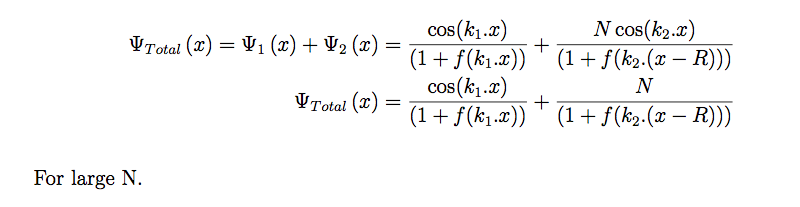# How did I correct Newton's and Gauss' LawsMERRY CHRISTMAS...:)

This is the correction proposed by the Hypergeometrical Universe Theory (HU).

Newton’s Law of Gravitation andGauss’ Law of Electrostatics.

HU proposes that the Universe is a lightspeed expanding hyperspherical hypersurface. This can be understood easily by just neglecting the hyper in the last frase.

3D Universe is a lightspeed expanding spherical surface

This is to emphasize that we are not in the “volume” of the Hypersphere. We inhabit a thin hypersurface. The radius of curvature of that surface (4D radius) is 13.58 billion light-years, thus very large.

In our neighborhood, that hypersphere can easily be approximated by a hyperplane.

This means that for short distances, we are in a moving hyperplanar frame of reference with an accommodating 4D velocity of light as $\sqrt2c$,

Equation(1)Under those conditions, the changing the velocity of the speed of light doesn’t do anything to the observable retarded potentials (Gravitational and Electrostatic).

The question is: What is really happening?

Are Gravitation and Electrostatic fields/force really decaying with the inverse of distance squared?

Measurements on short distances, would indicate that they certainly seem to be doing so.

Enters HU

The Hypergeometrical Universe Theory proposes that the dilaton field (from which HU derives Electrostatics and Gravitation) decays with the inverse of the number of cycles!!!!!! (not distance squared!!!!).

The dilaton field is given by:eq(1)

where k_1 is the k-vector (2pi/lambda1) associated with the metric waves of a single probe dilator. This probe dilator is the fundamental dilator (FD) , represented here in its Balls Diagram:where the lettering means parallel of perpendicular to our 3D Hyperspherical Hypersurface (our Universe).

FD is the basis for HU model for matter. In HU, matter is composed of space deformations, more specifically, matter is a polymer of FD, where FD is a coherence between stationary states of deformation of the local metric.The figure above represents the states involved. Since the proton has a larger area (area or footprint is proportional to inertial mass), it is being represented as an excited state of deformation. Polymeric coherences can be formed by adding 3D spatial rotations (either on the top level or on the bottom level). These coherence transmute electrons into protons or electron into positrons respectively.

As dilators transmute and travel with the hypersphere, they create the dilaton fields described by the equation (1).

Let’s nos consider a large mass or charge given by :The tolta dilaton field is given by:the approximation used was to make cos() =1 since as the number of dilators increase the peaks becomes too close and can be considered a continuous line (we become interested in the envelop of the dilaton field instead). f is equal to 1 except at the origin where f is such thatthat is, the dilaton field is emitted isotropically.

The sum of these fields is what defines the position of the dilator shown as x. x is the shift from one de Broglie step to the other due to interaction with the neighboring mass or charge.HU Quantum Lagrangian Principle (QLP) prescribes that dilators are lazy and will not do any work, that is, they will always dilate in phase with the surrounding field.This means the the derivative of the total field equated to zero will be solved to find where the maximum is. There is where the dilator will go.

The total dilaton field is:The derivative of the first term is just a derivative of a sin(x).This is the Grand Unification Equation!From it HU derives the Gyrogravitational Equation below:and the equivalent for Charge DilatorsThis equation indicates that the Gravitational constant G is inversely proportional to the 4D radius of the Universe. HU proposes a different way of peering into the past. We look across the 4D chasm (albeit through the prior hyperspheres).Observations indicate that light travels along the line-of-sight path. This means that light is emitted and travel layer by layer, slightly off 45 degrees. Remember that the Universe is 13.58 billion light-years wide and each de Broglie step is 0.19 femtometers, so there are many, many layers for the angle difference to be distributed.HU view of the Supernova explosions can be understood better here:The yellow scattered data are the Supernova explosions and the solid line are HU predictions. This is a model without a parameter.

For this to be correct, equation (1)has to be correct. This means that the Gravitational and Electrostatic fields are not directly dependent upon distances. They are dependent upon the number of cycles (de Broglie cycles) that the field has to traverse!

Any point without an inner hypersphere presents the same decay independently where they are physically located (independent upon the distance). The only thing that matters are the number of circles they cross.

This can be understood if one considers the decay of light. Maxwell equations explain to us that an electromagnetic field induces polarization which in turn creates electromagnetic field and so on and so forth.

HU polarizable media is the Universe and it travels radially always a speed c. This means that no matter where the k-vector points to, the radial velocity of light will always be c.

This also means that ANCIENT PHOTONS SLOW DOWN AS THEY APPROACH US.

IN SUMMARY:

The Hypergeometrical Universe Theory challenges Newton’s and Gauss’ equations by correcting their dependences into number of de Broglie cycles and not just plain distances.where the new denominator is the change in Cosmological Time (dimensionalized by multiplication by c).

This is the answer to how long-range Gravitation and Electromagnetism behave.

Current rating: 3.7

### New Comment

required

required (not published)

optional

required